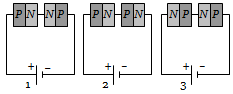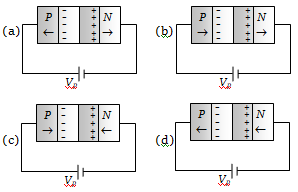Two PN-junctions can be connected in series by three different methods as shown in the figure. If the potential difference in the junctions is the same, then the correct connections will be(a) In the circuit (1) and (2)                    (b) In the circuit (2) and (3)
(c) In the circuit (1) and (3)                    (d) Only in the circuit (1)

Concept Questions :-

PN junction
High Yielding Test Series + Question Bank - NEET 2020

Difficulty Level:

In the depletion region of an unbiased P-N junction diode there are

(a) Only electrons

(b) Only holes

(c) Both electrons and holes

(d) Only fixed ions

Concept Questions :-

PN junction

Difficulty Level:

On increasing the reverse bias to a large value in a PN-junction diode, current

(a) Increases slowly                  (b) Remains fixed
(c) Suddenly increases              (d) Decreases slowly

Concept Questions :-

PN junction
High Yielding Test Series + Question Bank - NEET 2020

Difficulty Level:

In the case of forward biasing of PN-junction, which one of the following figures correctly depicts the direction of flow of carriersConcept Questions :-

PN junction
High Yielding Test Series + Question Bank - NEET 2020

Difficulty Level:

A semiconductor device is connected in a series circuit with a battery and a resistance. A current is found to pass through the circuit. If the polarity of the battery is reversed, the current drops almost to zero. The device may be

(a) A P-type semiconductor                (b) An N-type semiconductor
(c) A PN-junction                               (d) An intrinsic semiconductor

Concept Questions :-

PN junction
High Yielding Test Series + Question Bank - NEET 2020

Difficulty Level:

The approximate ratio of resistances in the forward and reverse bias of the PN-junction diode is

(a) ${10}^{2}:1$                     (b) ${10}^{-2}:1$
(c) $1:{10}^{-4}$                   (d) $1:{10}^{4}$

Concept Questions :-

PN junction
High Yielding Test Series + Question Bank - NEET 2020

Difficulty Level:

In a junction diode, the holes are due to

(a) Protons                               (b) Neutrons
(c) Extra electrons                     (d) Missing of electrons

Concept Questions :-

Types of semiconductors

Difficulty Level:

In forward bias, the width of potential barrier in a P-N junction diode

(a) Increases

(b) Decreases

(c) Remains constant

(d) First increases then decreases

Concept Questions :-

PN junction
High Yielding Test Series + Question Bank - NEET 2020

Difficulty Level:

The cause of the potential barrier in a P-N diode is

(a) Depletion of positive charges near the junction

(b) Concentration of positive charges near the junction

(c) Depletion of negative charges near the junction

(d) Concentration of positive and negative charges near the junction

Concept Questions :-

PN junction

Difficulty Level:

In a PN-junction diode not connected to any circuit

(a) The potential is the same everywhere

(b) The P-type is at higher potential than the N-type side

(c) There is an electric field at the junction directed from the N- type side to the P- type side

(d) There is an electric field at the junction directed from the P-type side to the N-type side

Concept Questions :-

PN junction